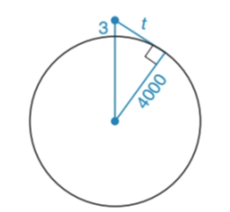Chapter 6.2, Problem 28EElementary Geometry For College St...

7th Edition
Alexander + 2 others
ISBN: 9781337614085

Solutions

Chapter
SectionElementary Geometry For College St...

7th Edition
Alexander + 2 others
ISBN: 9781337614085
Textbook Problem

From the veranda of a beachfront hotel, Manny is searching the seascape through his binoculars. A ship suddenly appears on the horizon. If Manny is 80     ft above the earth, how far is the ship out at sea?(HINT: See Exercise 27 and note that 1   m i = 5280 f t .)An airplane reaches an altitude of 3     mi above the earth. Assuming a clear day and that a passenger has binoculars, how far can the passengers see?(HINT: The radius of the earth is approximately 4000 mi.)To determine

To find:

The distance of the ship out at sea.

Explanation

Given:

Manny is 80ft above the earth.

Calculation:

Convert 80ft into mi.

We know that 1mi=5280ft.

1ft=15280mi

Therefore,

80ft=80×15280mi80ft=0.015mi

From the figure the earth radius is given as 4000mi

Still sussing out bartleby?

Check out a sample textbook solution.

See a sample solution

The Solution to Your Study Problems

Bartleby provides explanations to thousands of textbook problems written by our experts, many with advanced degrees!

Get Started

In Exercises 914, evaluate the expression. 14. 3543183

Applied Calculus for the Managerial, Life, and Social Sciences: A Brief Approach

Evaluate the indefinite integral. 2t2t+3dt

Single Variable Calculus: Early Transcendentals

True or False: x2+1x3dx may be solved using a trigonometric substitution.

Study Guide for Stewart's Single Variable Calculus: Early Transcendentals, 8th